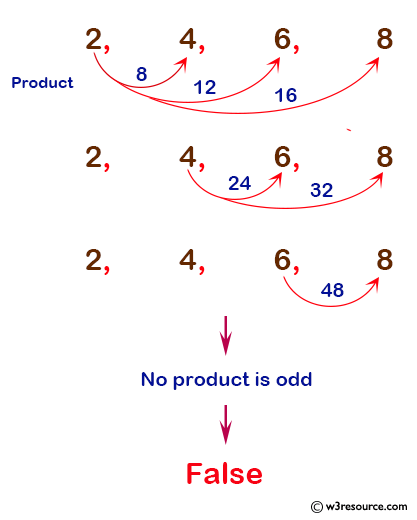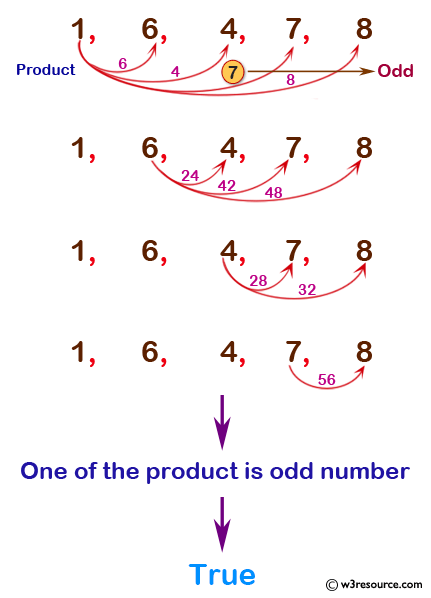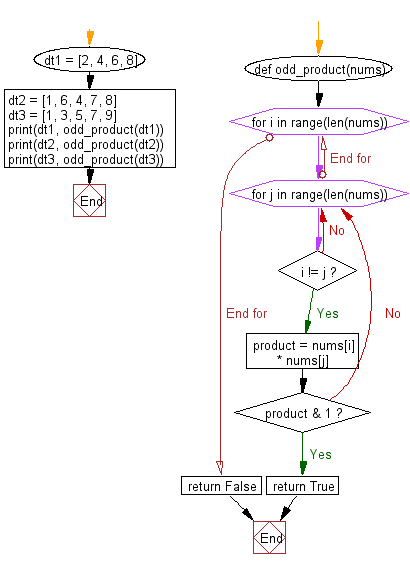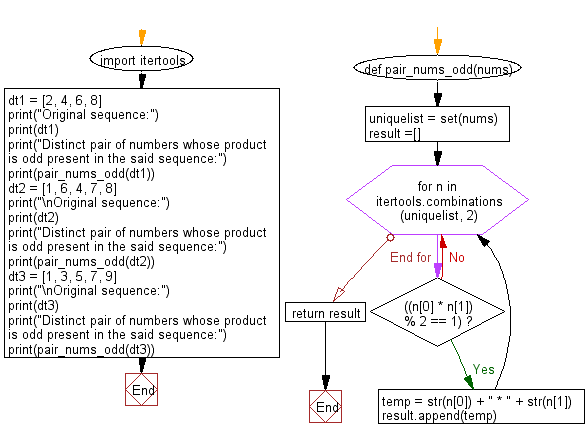﻿ Python: Check whether a distinct pair of numbers whose product is odd present in a sequence of integer values - w3resource# Python: Check whether a distinct pair of numbers whose product is odd present in a sequence of integer values

## Python Basic: Exercise-150 with Solution

Write a Python function to check whether a distinct pair of numbers whose product is odd present in a sequence of integer values.

Sample Solution:

Python Code :

``````def odd_product(nums):
for i in range(len(nums)):
for j in range(len(nums)):
if  i != j:
product = nums[i] * nums[j]
if product & 1:
return True
return False
dt1 = [2, 4, 6, 8]
dt2 = [1, 6, 4, 7, 8]
dt3 = [1, 3, 5, 7, 9]
print(dt1, odd_product(dt1));
print(dt2, odd_product(dt2));
print(dt3, odd_product(dt3));
``````

Sample Output:

```[2, 4, 6, 8] False
[1, 6, 4, 7, 8] True
[1, 3, 5, 7, 9] True
```

Pictorial Presentation of the sequence [2, 4, 6, 8]:Pictorial Presentation of the sequence [1, 6, 4, 7, 8]:Flowchart:## Visualize Python code execution:

The following tool visualize what the computer is doing step-by-step as it executes the said program:

Sample Solution:

Distinct pair of numbers whose product is odd present in a given sequence:

Python Code :

``````import itertools
def pair_nums_odd(nums):
uniquelist = set(nums)
result =[]
for n in itertools.combinations(uniquelist, 2):
if ((n * n) % 2 == 1):
temp = str(n) + " * " + str(n)
result.append(temp)
return result

dt1 = [2, 4, 6, 8]
print("Original sequence:")
print(dt1)
print("Distinct pair of numbers whose product is odd present in the said sequence:")
print(pair_nums_odd(dt1));
dt2 = [1, 6, 4, 7, 8]
print("\nOriginal sequence:")
print(dt2)
print("Distinct pair of numbers whose product is odd present in the said sequence:")
print(pair_nums_odd(dt2));
dt3 = [1, 3, 5, 7, 9]
print("\nOriginal sequence:")
print(dt3)
print("Distinct pair of numbers whose product is odd present in the said sequence:")
print(pair_nums_odd(dt3));
``````

Sample Output:

```Original sequence:
[2, 4, 6, 8]
Distinct pair of numbers whose product is odd present in the said sequence:
[]

Original sequence:
[1, 6, 4, 7, 8]
Distinct pair of numbers whose product is odd present in the said sequence:
['1 * 7']

Original sequence:
[1, 3, 5, 7, 9]
Distinct pair of numbers whose product is odd present in the said sequence:
['1 * 3', '1 * 5', '1 * 7', '1 * 9', '3 * 5', '3 * 7', '3 * 9', '5 * 7', '5 * 9', '7 * 9']
```

Pictorial Presentation of the sequence [1, 3, 5, 7, 9]:Flowchart:## Visualize Python code execution:

The following tool visualize what the computer is doing step-by-step as it executes the said program:

Python Code Editor :

Have another way to solve this solution? Contribute your code (and comments) through Disqus.

What is the difficulty level of this exercise?

Test your Python skills with w3resource's quiz

﻿

## Python: Tips of the Day

Unpack variables from iterable:

```# One can unpack all iterables (tuples, list etc)
>>> a, b, c = 1, 2, 3
>>> a, b, c
(1, 2, 3)

>>> a, b, c = [1, 2, 3]
>>> a, b, c
(1, 2, 3)
```